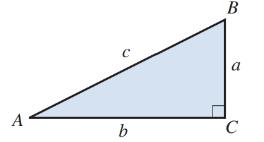# Using Illustration 1, find the measure of each acute angle for each right triangle: ILLUSTRATION 1 a = 25.45 in ., c = 41.25 in .### Elementary Technical Mathematics

12th Edition
Dale Ewen
Publisher: Cengage Learning
ISBN: 9781337630580### Elementary Technical Mathematics

12th Edition
Dale Ewen
Publisher: Cengage Learning
ISBN: 9781337630580

#### Solutions

Chapter
Section
Chapter 13.2, Problem 14E
Textbook Problem

## Using Illustration 1, find the measure of each acute angle for each right triangle:ILLUSTRATION 1 a = 25.45  in .,  c = 41.25  in .

Expert Solution
To determine

To calculate: The measure of the acute angle for a right angle with a=25.45 in. and c=41.25 in.

### Explanation of Solution

Given Information:

The right angled triangle has sides, a=25.45 in and c=41.25 in,

Formula used:

For a right angled triangle,

The trigonometric ratio for sine of angle A is

sinA=length of side opposite angle Alength of hypotenuse=ac

The angle sum of the acute angles of a right angled triangle is

A+B=90°

Calculation:

Consider the provided right angled triangle,

Apply the formula for sine of a right angled triangle, and substitute 25.45 for a and 41.25 for c,

sinA=25.4541.25=25454125=0

### Want to see the full answer?

Check out a sample textbook solution.See solution

### Want to see this answer and more?

Bartleby provides explanations to thousands of textbook problems written by our experts, many with advanced degrees!

See solution